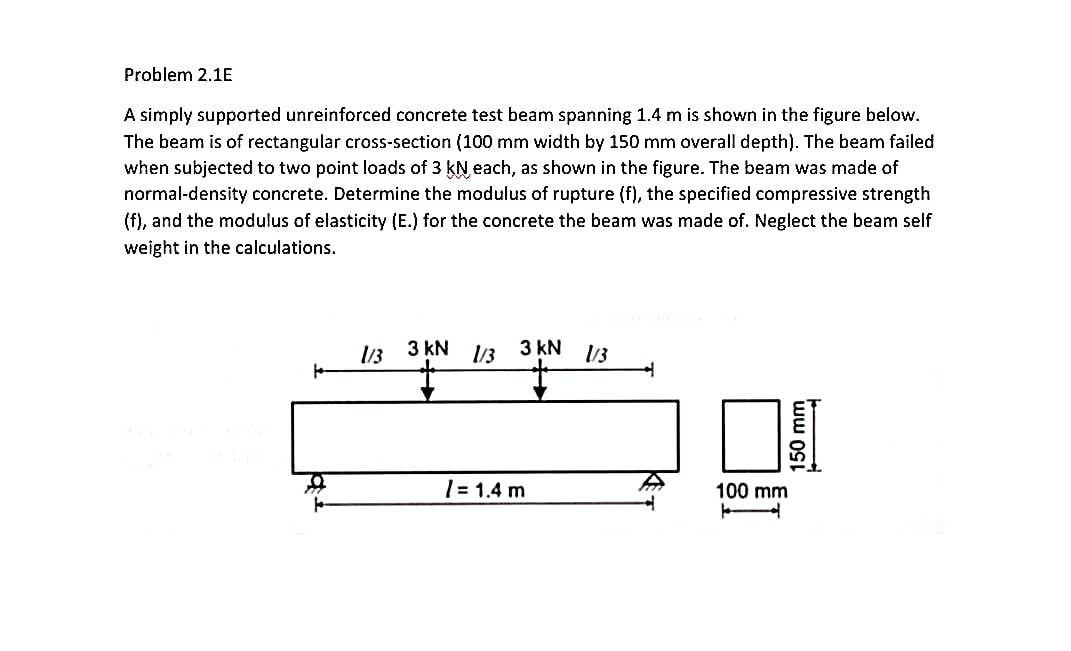# Question Problem 2.1E A simply supported unreinforced concrete test beam spanning 1.4 m is shown in the figure below. The beam is of rectangular cross-section (100 mm width by 150 mm overall depth). The beam failed when subjected to two point loads of 3 kN each, as shown in the figure. The beam was made of normal-density concrete. Determine the modulus of rupture (f), the specified compressive strength (f), and the modulus of elasticity (E.) for the concrete the beam was made of. Neglect the beam self weight in the calculations. 1/3 3 kN 13 3 kN 13 150 mm 1 = 1.4 m 100 mmA0UGTJ The Asker · Civil EngineeringTranscribed Image Text: Problem 2.1E A simply supported unreinforced concrete test beam spanning 1.4 m is shown in the figure below. The beam is of rectangular cross-section (100 mm width by 150 mm overall depth). The beam failed when subjected to two point loads of 3 kN each, as shown in the figure. The beam was made of normal-density concrete. Determine the modulus of rupture (f), the specified compressive strength (f), and the modulus of elasticity (E.) for the concrete the beam was made of. Neglect the beam self weight in the calculations. 1/3 3 kN 13 3 kN 13 150 mm 1 = 1.4 m 100 mm
More
Transcribed Image Text: Problem 2.1E A simply supported unreinforced concrete test beam spanning 1.4 m is shown in the figure below. The beam is of rectangular cross-section (100 mm width by 150 mm overall depth). The beam failed when subjected to two point loads of 3 kN each, as shown in the figure. The beam was made of normal-density concrete. Determine the modulus of rupture (f), the specified compressive strength (f), and the modulus of elasticity (E.) for the concrete the beam was made of. Neglect the beam self weight in the calculations. 1/3 3 kN 13 3 kN 13 150 mm 1 = 1.4 m 100 mm